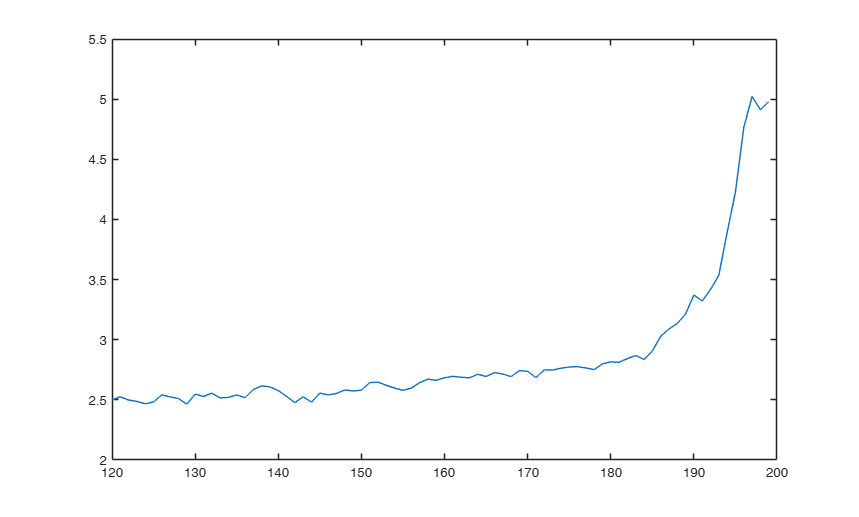FSMmmdeasy

FSMmmdeasy is exactly equal to FSMmmd but it is much less efficient

Syntax

• mmd=FSMmmdeasy(Y,bsb)example
• mmd=FSMmmdeasy(Y,bsb,Name,Value)example
• [mmd,Un]=FSMmmdeasy(___)example
• [mmd,Un,varargout]=FSMmmdeasy(___)example

Description

mmd =FSMmmdeasy(Y, bsb) Minimum Mahalanobis distance.

mmd =FSMmmdeasy(Y, bsb, Name, Value) FSMmmdeasy with optional arguments.

[mmd, Un] =FSMmmdeasy(___) Checking the unit(s) included in the subset at each step of the search.

[mmd, Un, varargout] =FSMmmdeasy(___) Checking the units belonging to subset in each step of the search.

Examples

expand allMinimum Mahalanobis distance.

Personalized initial subset (small n). Create an initial subset with the 4 observations which fell the smallest number of times outside the robust bivariate ellipses and with the lowest Mahalanobis Distance.

n=200;
v=3;
m0=4;
randn('state',123456);
Y=randn(n,v);
%Contaminated data
Ycont=Y;
Ycont(1:5,:)=Ycont(1:5,:)+3;
[fre]=unibiv(Y);
fre=sortrows(fre,[3 4]);
bs=fre(1:m0,1);
mmd=FSMmmdeasy(Ycont,bs);
plot(mmd(:,1),mmd(:,2))FSMmmdeasy with optional arguments.

Plotting the bandwith of the minimum Mahalanobis distance

n=200;
v=3;
m0=4;
randn('state',123456);
Y=randn(n,v);
%Contaminated data
Ycont=Y;
Ycont(1:5,:)=Ycont(1:5,:)+3;
[fre]=unibiv(Y);
fre=sortrows(fre,[3 4]);
bs=fre(1:m0,1);
[mmd]=FSMmmdeasy(Ycont,bs,'plots',1);Checking the unit(s) included in the subset at each step of the search.

% Un contains the unit(s) present in the new subset but not in the old one.
n=200;
v=3;
m0=4;
randn('state',123456);
Y=randn(n,v);
%Contaminated data
Ycont=Y;
Ycont(1:5,:)=Ycont(1:5,:)+3;
[fre]=unibiv(Y);
fre=sortrows(fre,[3 4]);
bs=fre(1:m0,1);
[mmd,Un]=FSMmmdeasy(Ycont,bs,'plots',1);

Checking the units belonging to subset in each step of the search.

Personalized initial subset (large n). Each row of BB matrix is associated to a unit while each colum is associated to a step of the fwd search.

n=500;
v=3;
m0=10;
randn('state',123456);
Y=randn(n,v);
% 25 per cent of Contaminated data
Ycont=Y;
Ycont(1:50,:)=Ycont(1:50,:)+3;
[fre]=unibiv(Y);
fre=sortrows(fre,[3 4]);
bs=fre(1:m0,1);
[mmd,Un,BB]=FSMmmdeasy(Ycont,bs,'plots',1);

Input Arguments

Y — Input data. Matrix.

n x v data matrix; n observations and v variables. Rows of Y represent observations, and columns represent variables.

Missing values (NaN's) and infinite values (Inf's) are allowed, since observations (rows) with missing or infinite values will automatically be excluded from the computations.

Data Types: single|double

bsb — Units forming subset. Vector.

List of units forming the initial subset.

If bsb=0 (default) then the procedure starts with p units randomly chosen else if bsb is not 0 the search will start with m0=length(bsb)

Data Types: single | double

Name-Value Pair Arguments

Specify optional comma-separated pairs of Name,Value arguments. Name is the argument name and Value is the corresponding value. Name must appear inside single quotes (' '). You can specify several name and value pair arguments in any order as Name1,Value1,...,NameN,ValueN.

Example: 'init',50 , 'plots',0 , 'msg',0

init —Point where to start monitoring required diagnostics.scalar.

Note that if bsb is supplied, init>=length(bsb). If init is not specified it will be set equal to floor(n*0.6).

Example: 'init',50

Data Types: double

plots —It specify whether it is necessary to produce the plots of minimum Mahalanobis distance.scalar.

If plots=1, a plot of the monitoring of minMD among the units not belonging to the subset is produced on the screen with 1% 50% and 99% confidence bands else (default) no plot is produced.

Example: 'plots',0

Data Types: double

msg —It controls whether to display or not messages about great interchange on the screen.scalar.

If msg==1 (default) messages are displyed on the screen else no message is displayed on the screen.

Example: 'msg',0

Data Types: double

Output Arguments

mmd —Monitoring of minimum Mahalanobis distance each step of the forward search. (n-init) -by- 2 matrix

1st col = fwd search index (from init to n-1).

2nd col = minimum Mahalanobis distance.

Un —Unit(s) included in the subset at each step of the search. (n-init) -by- 11 Matrix

REMARK: in every step the new subset is compared with the old subset. Un contains the unit(s) present in the new subset but not in the old one.

Un(1,2) for example contains the unit included in step init+1.

Un(end,2) contains the units included in the final step of the search.

varargout —Units belonging to subset in each step of the search. BB : n -by- (n-init+1) matrix

Each row is associated to a unit while each colum is associated to a step of the fwd search.

Atkinson, A.C., Riani, M. and Cerioli, A. (2004), "Exploring multivariate data with the forward search", Springer Verlag, New York.